# Introduction to modi

#### 2018-11-14

A first version of this vignette has been published in the Austrian Journal of Statistics (Bill and Hulliger 2016).

## Introduction

The distribution of multivariate quantitative survey data usually is not normal. Skewed and semi-continuous distributions occur often. In addition, missing values and non-response is common. All together this mix of problems makes multivariate outlier detection difficult. Examples of surveys where these problems occur are most business surveys and some household surveys like the Survey for the Statistics of Income and Living Condition (SILC) of the European Union. Several methods for multivariate outlier detection are collected in the R package modi. This vignette gives an overview of the package modi and its functions for outlier detection and corresponding imputation. The use of the methods is explained with a business survey data set. The discussion covers pre- and post-processing to deal with skewness and zero-inflation, advantages and disadvantages of the methods and the choice of the parameters.

## Overview

The following outlier detection and imputation functions are provided in modi:

• BEM() is an implementation of the BACON-EEM algorithm to detect outliers under the assumption of a multivariate normal distribution.
• TRC() is an implementation of the transformed rank correlation (TRC) algorithm to detect outliers.
• EAdet() is an outlier detection method based on the epidemic algorithm (EA).
• EAimp() is an imputation method based on the epidemic algorithm (EA).
• GIMCD() is an outlier detection method based on non-robust Gaussian imputation (GI) and the highly robust minimum covariance determinant (MCD) algorithm.
• POEM() is a nearest neighbor imputation method for outliers and missing values.
• winsimp() is an imputation method for outliers and missing values based on winsorization and Gaussian imputation.
• ER() is a robust multivariate outlier detection algorithm that can cope with missing values.

Furthermore, modi provides a set of utility functions:

• plotMD() is a function to plot the Mahalanobis distances.
• MDmiss() is a function to calculate Mahalanobis distances when missing values occur.
• weighted.quantile() is a function to calculate weighted quantiles.
• weighted.var() is a function to calculate weighted variances analogous to the weighted.mean() function in the stats package.

## Data

The modi package contains two datasets, first a version of the well known Bushfire dataset containing missing values (bushfirem), and second the sepe dataset which is an anonymized sample of a pilot survey on environment protection expenditures of the Swiss private economy (Federal Statistical Office). In this vignette, we will use the sepe dataset.

The units in the sepe dataset are enterprises and all monetary variables are in thousand Swiss Francs. The dataset contains 675 observations on 23 variables. However, we will only use the following variables:

• idnr: ID of the observation
• weight: sampling weight
• totinvwp: total investment in water protection measures
• totinvwm: total investment in waste management measures
• totinvap: total investment in air protection measures
• totinvto: overall total investment
• totexpwp: total expenditure in water protection measures
• totexpwm: total expenditure in waste management measures
• totexpap: total expenditure in air protection measures
• totexpto: overall total expenditure
idnr weight totinvwp totinvwm totinvap totinvto totexpwp totexpwm totexpap totexpto
15 25.3 NA NA 0 NA 40 NA 0 60
100 25.3 0 0 0 0 0 0 0 0
103 25.3 0 0 0 0 237 18 10 265
166 25.3 0 0 0 0 0 0 0 0
300 25.3 154 0 0 154 8 27 0 35
305 25.3 2 3 40 45 35 11 0 46
379 25.3 0 0 14 14 21 23 0 44
387 25.3 0 0 0 0 3 NA NA NA
535 25.3 0 0 0 20 0 6 0 6
603 25.3 30 0 100 180 4 11 0 15

The overall total investement and expenditure have been collected in the survey as well. Therefore, they do not always correspond to the sum over the individual investments and expenditures. The dataset contains 677 items with missing values and 2’595 items with a value of zero. Since some of the functions in this package relie on the assumption of a multivariate normal distribution, the zeros are declared as missing values.

# attach data
data("sepe")

# recode 0s as NA
sepenozero <- sepe
sepenozero[sepenozero == 0] <- NA

The resulting data is highly asymmetric and we thus apply the following logarithmic transformation log(x + 1).

# relevant variables
sepevar8 <- c("totinvwp","totinvwm","totinvap","totinvto",
"totexpwp","totexpwm","totexpap","totexpto")

# log(x + 1) transformation
sepe_transformed <- log(sepenozero[ ,sepevar8] + 1)

# show the first 5 observations
#>   totinvwp totinvwm totinvap totinvto totexpwp totexpwm totexpap totexpto
#> 1       NA       NA       NA       NA 3.713572       NA       NA 4.110874
#> 2       NA       NA       NA       NA       NA       NA       NA       NA
#> 3       NA       NA       NA       NA 5.472271 2.944439 2.397895 5.583496
#> 4       NA       NA       NA       NA       NA       NA       NA       NA
#> 5 5.043425       NA       NA 5.043425 2.197225 3.332205       NA 3.583519
#> 6 1.098612 1.386294 3.713572 3.828641 3.583519 2.484907       NA 3.850148

## How to apply functions in the modi package

In the following sections, we introduce the different outlier detection and imputation functions in the modi package. All functions are illustrated with examples based on the sepe dataset.

### BEM() and Winsimp()

BEM() is an implementation of the BACON-EEM algorithm that was developed by (Béguin and Hulliger 2008). The BACON-EEM algorithm starts with a small subset of good observations, that are not outliers. Then, the mean and the covariance matrix of this subset are estimated with the EM-algorithm. The estimates take the sample design into account. Then, BEM() computes the Mahalonobis distance (MD) for all data points. Observations that are below the cutpoint defined by a $$\chi^2$$-quantile are the new good subset. The algorithm iterates until convergence. Important control parameters in BEM() are the size of the initial good subset as a multiple c0 of the dimension of the data (number of columns) and the probability alpha to determine the quantile of the $$\chi^2$$-distribution for the cutpoint.

The default value for the initial good subset is c0 = 3. However, in our case this results in a too small initial subset with many missing values and hence, the covariance matrix used to calculate the Mahalanobis distances is singular. Therefore, we set c0 = 5 which results in a size of the initial good subset of 40 observations.

# run the BEM() algorithm
res.bem <- BEM(sepe_transformed, sepe$weight, c0 = 5) #> Warning: missing observations 2 4 11 12 41 57 60 65 67 68 70 90 103 105 106 107 108 109 110 114 118 125 129 132 135 136 144 166 178 181 182 188 193 208 209 210 214 215 216 218 219 223 237 238 244 245 256 259 260 261 267 268 269 270 272 274 276 277 278 279 281 301 304 312 313 316 321 323 327 332 334 337 339 346 348 350 351 354 357 374 375 392 395 397 401 403 409 410 411 412 417 437 439 450 451 466 467 469 471 473 474 479 492 493 494 496 499 500 505 509 510 518 523 525 526 528 529 530 538 545 546 547 549 550 551 552 553 554 556 558 559 564 569 571 572 574 575 604 605 618 619 628 629 630 632 637 638 644 646 648 655 656 657 659 661 662 665 666 removed from the data #> #> BEM has detected 385 outlier(s) in 0.82 seconds. #>  We can immediately see that a lot (158 to be precise) of the observations have been removed by BEM() because they are completely missing. BEM() identifies 385 of the remaining 517 observations as outliers. This is obviously implausible and happens if the default value 0.01 for the parameter representing the cut-off quantile, alpha, is a bad choice. Therefore, it is generally a good idea to decrease alpha. A good rule of thumb is to divide the default value (0.01) by the number of observations (alpha = 0.01 / nrow(data)) if the number of observations is above 100. # run the BEM() algorithm with different alpha res.bem <- BEM(sepe_transformed, sepe$weight, c0 = 5, alpha = 0.01 / nrow(sepe_transformed))
#> Warning: missing observations 2 4 11 12 41 57 60 65 67 68 70 90 103 105 106 107 108 109 110 114 118 125 129 132 135 136 144 166 178 181 182 188 193 208 209 210 214 215 216 218 219 223 237 238 244 245 256 259 260 261 267 268 269 270 272 274 276 277 278 279 281 301 304 312 313 316 321 323 327 332 334 337 339 346 348 350 351 354 357 374 375 392 395 397 401 403 409 410 411 412 417 437 439 450 451 466 467 469 471 473 474 479 492 493 494 496 499 500 505 509 510 518 523 525 526 528 529 530 538 545 546 547 549 550 551 552 553 554 556 558 559 564 569 571 572 574 575 604 605 618 619 628 629 630 632 637 638 644 646 648 655 656 657 659 661 662 665 666 removed from the data
#>
#>  BEM has detected 89 outlier(s) in 0.53 seconds.
#> 

Now, the algorithm returns 89 outliers. Clearly, this is a more plausible result than before. With the help of PlotMD(), we can create a QQ-plot of the Mahalanobis distances and the corresponding F-distribution. As can be seen below, the minimal (squared) MD of the outliers is 37.14. However, by visual inspection of the QQ-plot we can see that a higher cutpoint would fit the data better.

# QQ-plot of MD vs. F-distribution
PlotMD(res.bem$dist, ncol(sepe_transformed), alpha = 0.95)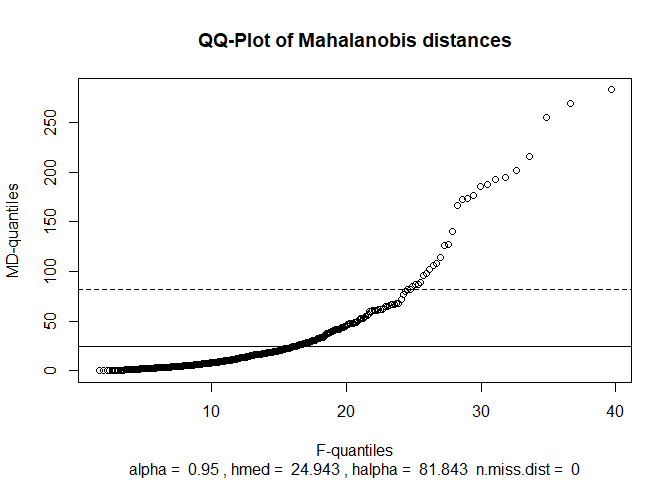# find the cutpoint chosen by BEM() min(res.bem$dist[res.bem$outind]) #>  37.14862 There are two ways of finding a better cutpoint: • Find the cutpoint by visually inspecting the distribution plot shown above. The cutpoint should be chosen such that it corresponds to the point where the distribution changes substantially. In our case, we could try the cutpoint 70 which results in 31 outliers. # find outliers with cutpoint 70 outind <- ifelse(res.bem$dist > 70, TRUE, FALSE)

# set NAs to FALSE
outind[is.na(outind)] <- FALSE

# how many outliers are there?
sum(outind)
#>  31
• The second option is to declare a specific fraction of observations to be outliers. For example, if we assume that 5% (or 26) of the observations are outliers, the cutpoint for the (squared) Mahalanobis distances is 82.53.
# find cutpoint with fixed number of outliers
quantile(res.bem$dist, 0.95, na.rm = TRUE) #> 95% #> 82.52671 The following plot shows total investment and total expenditure (log-transformed) for every observation. The 89 Outliers found by BEM() are depicted as red crosses whereas the 31 outliers found by choosing the cutpoint according to a visual inspection of the QQ-plot are depicted as blue rectangles. Note that we can only plot two variables (total investment and expenditure) and thus, some outliers contained in the bulk of data may not look like outliers. # create transformed data including zeros df <- log(sepe[, sepevar8] + 1) # set up scatterplot totexpto vs. totinvto plot(df$totinvto, df$totexpto, type = "n", xlab = "Total Inv.", ylab = "Total Exp.") # plot comparison of outliers determined with visual cutpoint and default cutpoint points(df$totinvto[!res.bem$outind], df$totexpto[!res.bem$outind]) points(df$totinvto[res.bem$outind], df$totexpto[res.bem$outind], pch = 4, col = "red") points(df$totinvto[outind], df$totexpto[outind], pch = 0, col = "blue")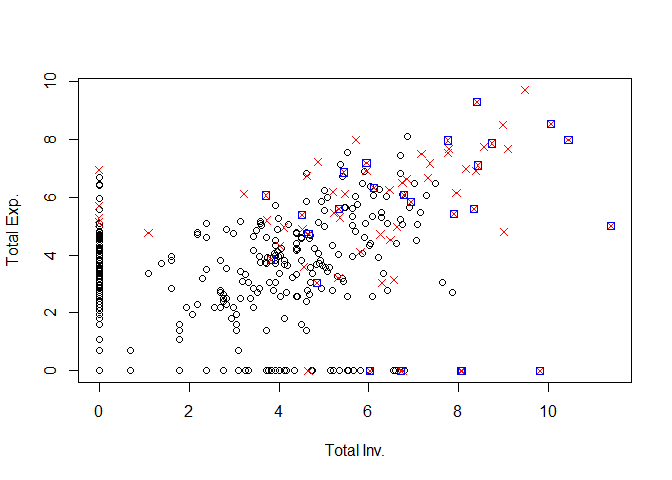After outliers have been detected, we can impute values. Here, we do this with Winsimp() which corresponds to a winsorisation (Wins) of outliers according to the Mahalanobis distance followed by an imputation (imp) under the multivariate normal model. We use the center and scatter calculated with BEM(). # apply Winsimp() res.winsimp <- Winsimp(sepe_transformed, res.bem$output$center, res.bem$output$scatter, outind) Here, we re-insert the zeros after the imputation since the zeros did not contribute to the multivariate normal model used for detection. Of course, it would also be possible to re-insert zeros before the imputation which might result in somewhat more coherent imputations. # get the imputed data imp_data <- res.winsimp$imputed.data

# indicate the zeros in original dataset
zeros <- ifelse(sepe[ ,sepevar8] == 0, 1, 0)

# redefine NAs as 0
zeros[is.na(zeros)] <- 0

# re-insert zeros
imp_data <- as.data.frame(ifelse(zeros == 1, 0, imp_data))

The following plot shows the outliers (log-transformed) with blue rectangles as well as the imputed values with green rectangles.

# create transformed data including zeros
df <- log(sepe[, sepevar8] + 1)

# set up scatterplot totexpto vs. totinvto
plot(df$totinvto, df$totexpto, type = "n", xlab = "Total Inv.", ylab = "Total Exp.")

# plot comparison of outliers determined with visual cutpoint and default cutpoint
points(df$totinvto[outind], df$totexpto[outind], pch = 0, col = "blue")
points(imp_data$totinvto[outind], imp_data$totexpto[outind], pch = 0, col = "green")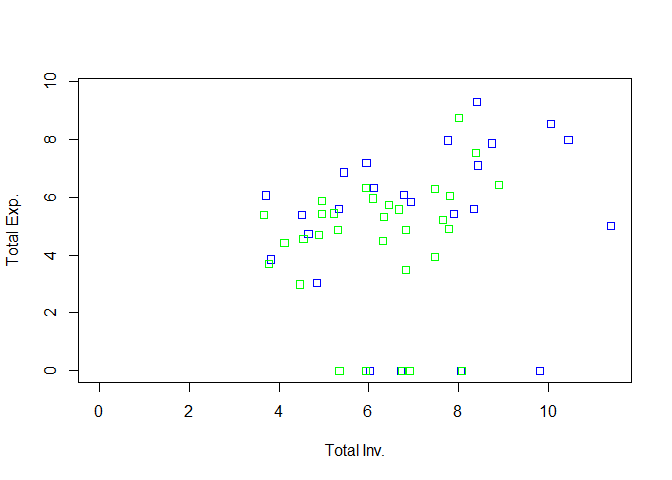### TRC()

TRC() is an implementation of the transformed rank correlation algorithm described in (Béguin and Hulliger 2004). The algorithm uses an orthogonal transformation of the data into the space of eigenvectors. Then, the center and scatter are recalculated with robust univariate estimators (median and median absolute deviation). Since these transformations require a complete dataset, the algorithm provisionally imputes missing values based on the best simple robust regression. Important control parameters of TRC() are gamma, which defines the minimal proportion of observations needed to determine an imputation model, and mincorr, which is the minimal correlation required for a regressor to be part of the provisional imputation model.

First, we create the original transformed dataset again, where all zeros are set to missing. This is useful as TRC relies on the assumption of multivariate normal data too.

# log(x + 1) transformation
sepe_transformed <- log(sepenozero[ ,sepevar8] + 1)

Using the default parameters results in TRC() finding 147 outliers. However, the default value gamma = 0.5 seems to be too high because most regressors for the provisional imputation of missing values have a slope of 0 (check output by adding monitor = TRUE). Hence, we decrease the value of gamma to 30 observations.

# run the TRC() algorithm
res.trc <- TRC(sepe_transformed, sepe$weight) #> Warning: missing observations 2 4 11 12 41 57 60 65 67 68 70 90 103 105 106 107 108 109 110 114 118 125 129 132 135 136 144 166 178 181 182 188 193 208 209 210 214 215 216 218 219 223 237 238 244 245 256 259 260 261 267 268 269 270 272 274 276 277 278 279 281 301 304 312 313 316 321 323 327 332 334 337 339 346 348 350 351 354 357 374 375 392 395 397 401 403 409 410 411 412 417 437 439 450 451 466 467 469 471 473 474 479 492 493 494 496 499 500 505 509 510 518 523 525 526 528 529 530 538 545 546 547 549 550 551 552 553 554 556 558 559 564 569 571 572 574 575 604 605 618 619 628 629 630 632 637 638 644 646 648 655 656 657 659 661 662 665 666 removed from the data #> #> Number of missing items: 2008 , percentage of missing items: 0.4854932 #> #> TRC has detected 147 outlier(s) in 0.11 seconds. Reducing gamma results in a better provisional imputation. However, the number of outliers stays almost the same (146 outliers). # run the TRC() algorithm res.trc <- TRC(sepe_transformed, sepe$weight, gamma = 30 / res.trc$output$sample.size)
#> Warning: missing observations 2 4 11 12 41 57 60 65 67 68 70 90 103 105 106 107 108 109 110 114 118 125 129 132 135 136 144 166 178 181 182 188 193 208 209 210 214 215 216 218 219 223 237 238 244 245 256 259 260 261 267 268 269 270 272 274 276 277 278 279 281 301 304 312 313 316 321 323 327 332 334 337 339 346 348 350 351 354 357 374 375 392 395 397 401 403 409 410 411 412 417 437 439 450 451 466 467 469 471 473 474 479 492 493 494 496 499 500 505 509 510 518 523 525 526 528 529 530 538 545 546 547 549 550 551 552 553 554 556 558 559 564 569 571 572 574 575 604 605 618 619 628 629 630 632 637 638 644 646 648 655 656 657 659 661 662 665 666 removed from the data
#>
#>  Number of missing items:  2008 , percentage of missing items:  0.4854932
#>
#>  TRC has detected 146 outlier(s) in 0.08 seconds.

The cutpoint chosen by TRC() is at 54.67. However, visual inspection of the QQ-plot below indicates that the cutpoint should be chosen at about 210.

# find the cutpoint chosen by TRC()
min(res.trc$dist[res.trc$outind])
#>  54.66964
# QQ-plot of MD vs. F-distribution
PlotMD(res.trc$dist, ncol(sepe_transformed))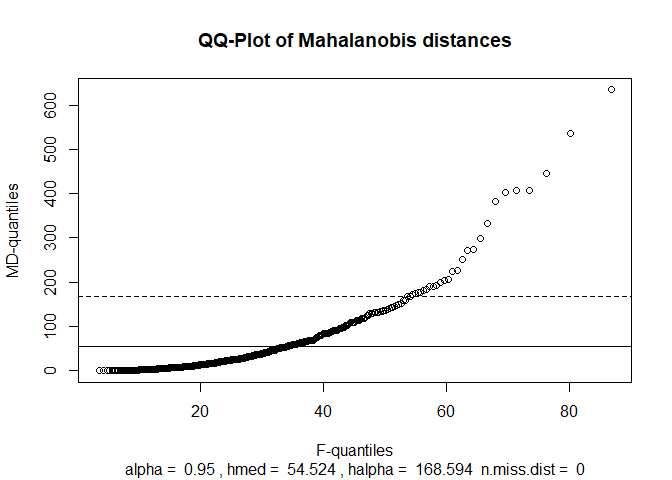Using 210 as the cutpoint results in 14 outliers. # find outliers with cutpoint 70 outind <- ifelse(res.trc$dist > 210, TRUE, FALSE)

# set NAs to FALSE
outind[is.na(outind)] <- FALSE

# how many outliers are there?
sum(outind)
#>  14

For the imputation of outliers, we can use Winsimp() as shown above in the section about BEM().

### GIMCD()

GIMCD() is an implementation of a non-robust Gaussian imputation (GI), followed by a robust minimum covariance determinant (MCD) to detect outliers. Note that GIMCD(), in contrast to BEM() and TRC(), imputes values for completely missing observations. Unfortunately, the algorithm cannot take weights into account. The only control parameter of the function GIMCD() is alpha which determines the quantile for the cutpoint. Its default value is 0.05. The parameters seedem and seedmcd are seed values for random number generators used in the algorithm. We set them here so that our results are reproducible.

# run the GIMCD() algorithm
res.gimcd <- GIMCD(sepe_transformed, seedem = 234567819, seedmcd = 4097)
#> GIMCD has detected 57 outliers in 1.03 seconds.

The output above shows that GIMCD() finds 57 outliers. The default cutpoint is at 16.49.

# find the cutpoint chosen by GIMCD()
min(res.gimcd$dist[res.gimcd$outind])
#>  16.49471

A visual inspection of the QQ-plot below shows that 24 might be a cutpoint that fits the data better.

# QQ-plot of MD vs. F-distribution
PlotMD(res.gimcd$dist, ncol(sepe_transformed))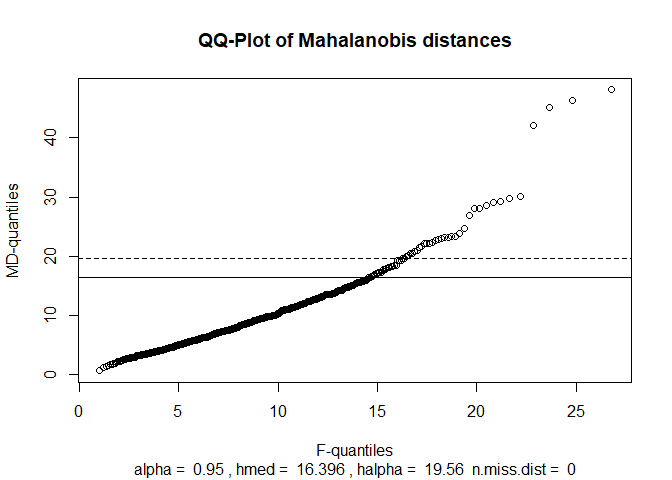Using 24 as the cutpoint results in 13 outliers. As before, we can use Winsimp() to impute values for the outliers. # find outliers with cutpoint 70 outind <- ifelse(res.gimcd$dist > 24, TRUE, FALSE)

# set NAs to FALSE
outind[is.na(outind)] <- FALSE

# how many outliers are there?
sum(outind)
#>  13

### EAdet() and EAimp()

The Epidemic Algorithm (EA) is implemented in two functions: EAdet() contains the detection algorthm and EAimp() contains the imputation algorithm. The details of EA are described in (Béguin and Hulliger 2004). Compared to the methods shown above, the Epidemic Algorithm does not rely on a distributional assumption. The basic idea of the Epidemic Algorithm is to simulate an epidemic that starts at a central point (a spatial median) and then infects points in the neighbourhood in a stepwise manner (check the documentation for details). The last infected points are nominated as outliers.

We can feed the original (log-transformed) sepe dataset to the detection function EAdet() in spite of the 48% of items with value zero.

# create transformed data including zeros
df <- log(sepe[, sepevar8] + 1)

# run the EAdet() algorithm
res.eadet <- EAdet(df, sepe$weight) #> #> Some mads are 0. Standardizing with 0.9 quantile absolute deviations!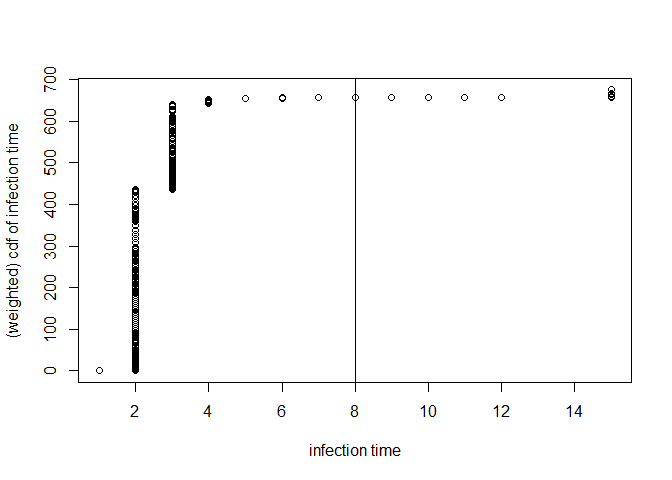#> #> EA detection has finished with 664 infected points in 0.08 seconds. # how many outliers? sum(res.eadet$outind, na.rm = TRUE)
#>  7

For the sepe data, the default cutpoint results in only 7 outliers. However, there is a further set of 11 observations that have not been infected. This may happen because they have too many missing values or because they are too far outlying. The function value outind is a logical vector with TRUE for the outliers (late infected), FALSE for the good observations (early infected), and NA for the never infected observations. In this example, the 11 not infected observations consist entirely of missing values.

By default, the (weighted) cumulative distribution function of the infection times is plotted. As before, selecting a good cutpoint needs some care. The default outlier rule declares observations that are infected at time 8 or later as outliers. Looking at the (weighted) cumulative distribution function of the infection times, it seems more reasonable to set the cutpoint to 5. Using this new cutpoint yields 20 outliers. The Epidemic Algorithm results in substantially less outliers than the other algorithms shown above.

# determine outliers based on new cutpoint
outind <- res.eadet$infection.time >= 5 # how many outliers are there? sum(outind, na.rm = TRUE) #>  20 The Epidemic Algorithm suffers from the many zeros and missing values in the sepe dataset because the inter-point distances are calculated on the basis of the jointly observed values only. Thus, this algorithm might be applied with care if your data contains many missing values. Of course, it is possible to discard completely missing observations (you may set the parameter rm.missing = TRUE) and it is also possible to set zeros to missing values before running EAdet(). We use EAimp() to impute values. Since the zeros were not set to missing, re-insertion is not an issue. EAimp() uses the distances calculated in EAdet() and starts an epidemic at each observation to be imputed until donors for the missing values are infected. Then a donor is selected randomly. # determine outliers based on new cutpoint res.eaimp <- EAimp(df, sepe$weight, outind = res.eadet$outind, duration = res.eadet$output\$duration)
#> Missing values in outlier indicator set to FALSE.
#>
#>  Dimensions (n,p): 675 8
#>  Number of complete records  449
#>  Number of records with maximum p/2 variables missing  633
#>  Number of imputands is  230
#>  Reach for imputation is  max
#>
#>  Number of remaining missing values is  0

## Conclusion

Multivariate outlier detection starts before running any outlier detection algorithms such as the ones implemented in the modi package. Every data set has its unique issues which need to be solved before outlier detection. Balance rules, missing value patterns as well as distributions need to be checked. For example, the sepe dataset has a zero inflated distribution and hence needs to be transformed in order to satisfy the distributional assumptions of the parametric algorithms. Once the assumptions are satisfied, the parameters of the outlier detection function need to be chosen.

Although the algorithms in the package modi have a high power of detecting multivariate outliers, user-intervention to choose the cutpoint is necessary as has been shown in the examples above. Moreover, it is important to check the results of the imputation. For example, we sometimes need to restrict imputed data to positive values.

Choosing an appropriate outlier detection method is difficult since all presented methods have advantages and disadvantages. The distribution of the sepe dataset is far from multivariate normal. Nevertheless, methods with an underlying assumption of multivariate normality may return satisfactory results if the distribution is uni-modal apart from the zero-inflation which must be treated before applying the outlier detection.